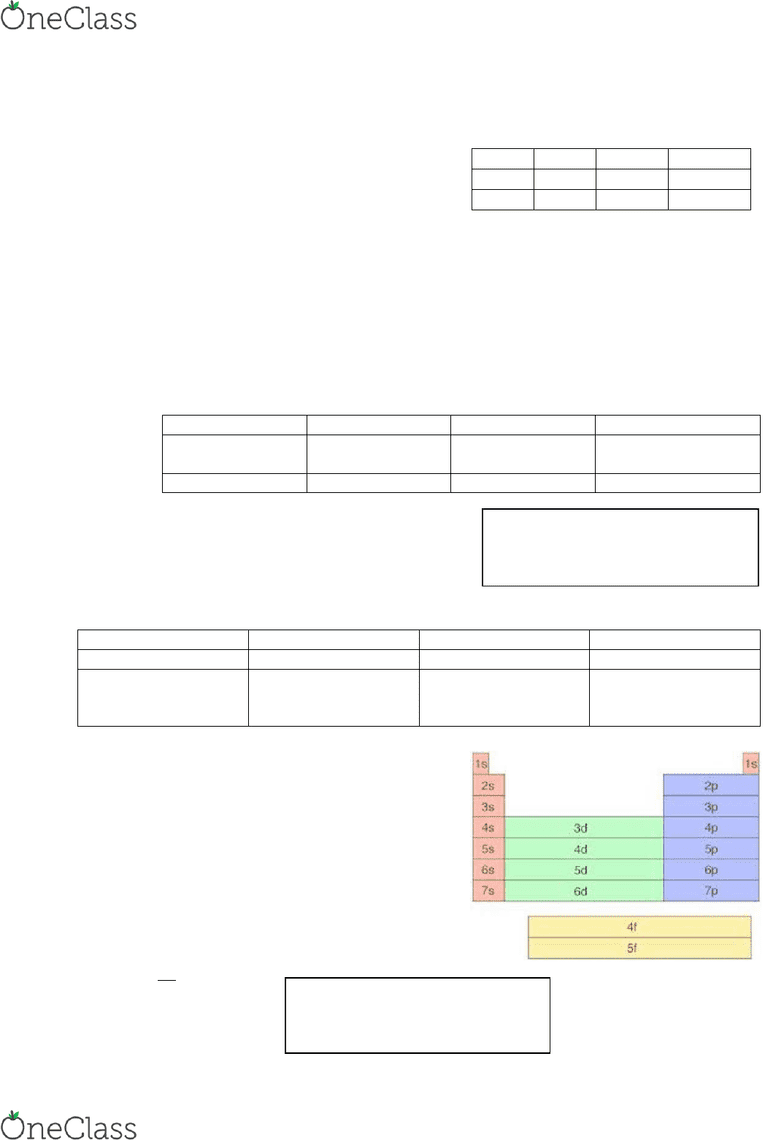Study Guides (390,000)
CA (150,000)
Queen's (5,000)
CHEM (80)
Final

# CHEM 112 Final: Exam Notes

by Kate

Department
Chemistry
Course Code
CHEM 112
Professor
John Carran
Study Guide
Final

This preview shows pages 1-3. to view the full 11 pages of the document.1
Electrons in Atoms
- energy produced by an oscillating electrical and magnetic field through waves, transmitted
by photons
- All types of electromagnetic radiant move through a vacuum at the speed of light
Frequency (inversely proportional to wavelength)- number of complete wavelengths that pass a
given point in one second (Hz)
Atomic Spectra
- high excited state low excited state: emission ΔE negative
- Low excited state high excited state: absorption ΔE positive)
Balmer Series- a set of spectral lines characteristic to hydrogen
Energy ∝ frequency ∝
Photoelectric effect- when light strikes the surface of a metal and electrons are ejected
- Occurs when the frequency of incident light exceeds particular threshold value
o If v < vo, then light intensity has no effect



Bohr Atom
- Atomic model for hydrogen-like species
- Lyman series, when nf = 1
- Balmer series, when nf = 2
- Paschen series, when nf = 3

Wave Mechanics
- Schrodinger equation (Ψ = EΨ
o Probability of finding an electron is zero at a node
Quantum
Principle Quantum #, n
- Specific energy level of orbital and it’s relative size
o Higher the n-value, higher the energy level larger the size of orbital
Secondary Quantum #, l
n = ,,…
ao = 0.53 Å
Z = atomic number
RH = 2.179 × 10-18 J
find more resources at oneclass.com
find more resources at oneclass.com

Only pages 1-3 are available for preview. Some parts have been intentionally blurred.2
- Represents energy subshell within each principle energy level
- Describes orbital shape
- Positive integer ranging from 0 to n-1
o s2 p6 d10 f14
- l = 0, s orbital, spherical shape
- l = 1, p orbital, peanut shape
- l = 2, d orbital, daisy shape
- l = 3, f orbital, f _____shape
Magnetic Quantum #, ml
- the orientation of the orbital in the space around the nucleus
- the integer with values ranging from l to +l including 0
o Ex. If l = 0, then ml can only be 0, therefore only one orientation is possible. This
makes sense since l = 0 has a spherical shape
o If l = I, then ml can either be -1, 0, or +1. This means the peanut-shaped orbital can
have three different orientations
Spin Quantum #, ms
- Describe how the electrons spin about their axes
as they move throughout the volume of their
atoms
)n Short…
N
L
ml
ms
Size or orbital
Shape of orbital
Orientation of orbital
Spin of electron
1, 2, 3, 4, 5, 6, 7
0 to n-1
-l, 0, +l
-½ (counter-
clockwise) or +½
(clockwise)
Aufbau Principle- fill up lower energy levels first
(und’s Principle- fill up all of the energy levels first
before doubling the electrons
Pauli Exclusion- No element can have the same four
quantum numbers
Heisenberg Uncertainty Principle


l = 0 (s)
l = 1 (p)
l = 2 (d)
ml= 0
1 #
ml= -1, 0, 1
 #’s
ml= -2, -1, 0, 1, 2
5 #’s
1 orientation
3 orientations
5 orientations
n = 1
n = 2
n = 3
n = 4
l = 0
l = 0,1
l = 0,1,2
l = 0,1,2,3
S
sp
spd
spdf
The value of ms can either be ½ or +½
Paired = -½ Unpaired = +½
Δp = oetu = u
Δx = positio
h = Planks constant (6.626 × 10-34 Js)
find more resources at oneclass.com
find more resources at oneclass.com

Only pages 1-3 are available for preview. Some parts have been intentionally blurred.3
Angular- number of cross sections
Total # of nodes = n-1
Trends in the Periodic Table
- As you go down the periodic table, you add energy levels makes radius increase
- As you go from left to right, adding electrons AND protons, increases attraction makes
Ions
Cations = # protons > # electrons
Anions = # protons < # electrons
Ionization Energy
- Energy required to remove one mole of electron from one mole of atoms or ions in its
GASEOUS state (endothermic = positive values)
o If IE high = hard to remove electron
o If IE low = easy to remove electron
As atom gets smaller. Attraction forces increases, making it harder to
remove one electron
Second IE is always greater than the first
Electron Affinity
- Difference in energy when an atom absorbs an electron (exothermic = negative values)
Diamagnetic- all electrons are paired
Paramagnetic- has unpaired electron
Chemical Bonding
Formal charge: # valence electrons - # bonds - # of unpaired electrons
Resonance structure- found when loecules have more than one possible Lewis structure
- Designated by a double-headed arrow and occurs from electron movement
1 cm = 10-2 m
 μ =  icroeter = -6 m
1 nm = 1 nanometer = 10-9 m
1 Å = angstrom = 10-10 m
find more resources at oneclass.com
find more resources at oneclass.com